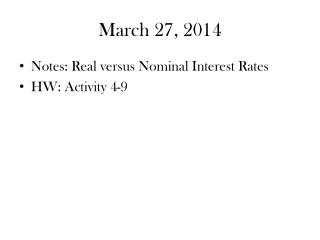DownloadDownload PresentationMarch 27, 2014

# March 27, 2014

Télécharger la présentation## March 27, 2014

- - - - - - - - - - - - - - - - - - - - - - - - - - - E N D - - - - - - - - - - - - - - - - - - - - - - - - - - -
##### Presentation Transcript

1. March 27, 2014 • Notes: Real versus Nominal Interest Rates • HW: Activity 4-9

2. Real versus Nominal Interest Rates • Intro Q: You bought a 1 year bond for \$1,000 and the bond paid an interest rate of 10%, at the end of the year would you be 10% wealthier? • What if the inflation rate was 8% • You would only be 2% wealthier! • What if the inflation rate was 12%? • You would be able to buy 2% less!

3. Real versus Nominal Interest Rates • NOMINAL INTEREST RATE: Interest rate before taking inflation into account (the rate quoted in loan and deposit agreements) • But to avoid purchasing power erosion through inflation… • REAL INTEREST RATE: interest rate that has been adjusted to remove the effects of inflation to reflect the real cost of funds to the borrower and the real yield to the lender.  • Expresses the change in your purchasing power! • The Real Interest Rate is the increase in purchasing power the lender wants to receive to forgo consumption now for consumption in the future. • The expected real interest rate represents the amount you need to receive in real terms to forgo consumption now for consumption in the future.

4. Real versus Nominal Inflation Rates • The Fisher Equation shows relationship between the Nominal Interest Rate, the Real Interest Rate, and the Inflation Rate: • r = i – • Obviously banks and customers do not know what inflation is going to be, so expected real interest rate is: • re = i - e • A bank sets the nominal interest rate equal to its expected real interest rate + expected inflation rate: • i = re + e

5. Real versus Nominal Interest Rates • If the Fed increases MS, PL increases… • If the Fed decreases MS, PL decreases… • In the SR, increases in MS decrease Nominal Interest Rate and Real Interest Rate (and vice versa) • In the LR, an increase in the MS will increase the PL and the Nominal Interest Rate.